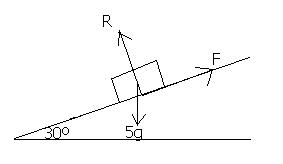## The Coefficient of Friction

Friction is "the resistance an object encounters in moving over another" (OED).

It is easier to drag an object over glass than sandpaper. The reason for this is that the sandpaper exerts more frictional resistance. In many problems, it is assumed that a surface is "smooth", which means that it does not exert any frictional force. In real life, however, this wouldn't be the case. A "rough" surface is one which will offer some frictional resistance.

Limiting Equilibrium

Imagine that you are trying to push a book along a table with your finger. If you apply a very small force, the book will not move. This must mean that the frictional force is equal to the force with which you are pushing the book. If the frictional force were less that the force produced by your finger, the book would slide forward. If it were greater, the book would slide backwards.

If you push the book a bit harder, it would still remain stationary. The frictional force must therefore have increased, or the book would have moved. If you continue to push harder, eventually a point is reached when the frictional force increases no more. When the frictional force is at its maximum possible value, friction is said to be limiting. If friction is limiting, yet the book is still stationary, it is said to be in limiting equilibrium. If you push ever so slightly harder, the book will start to move. If a body is moving, friction will be taking its limiting value.

In summary:

The frictional force between two objects is not constant, but increases until it reaches a maximum value. When the frictional force is at its maximum, the body in question will either be moving or will be on the verge of moving.

The Coefficient of Friction

The coefficient of friction is a number which represents the friction between two surfaces. Between two equal surfaces, the coefficient of friction will be the same. The symbol usually used for the coefficient of friction is m

The maximum frictional force (when a body is sliding or is in limiting equilibrium) is equal to the coefficient of friction × the normal reaction force.

• F = mR

Where m is the coefficient of friction and R is the normal reaction force.

This frictional force, F, will act parallel to the surfaces in contact and in a direction to oppose the motion that is taking/ trying to take place.

Example

A particle of mass 5 kg is at limiting equilibrium on a rough plane which is inclined at an angle of 30 degrees to the horizontal. Find the coefficient of friction between the particle and the plane.Resolving up the plane:
F - 5gsin30 = 0

Resolving perpendicular to the plane:
R = 5gcos30

In limiting equilibrium, so F = mR
5gsin30 = m5gcos30

m = sin30/cos30 = 0.577 (3sf)